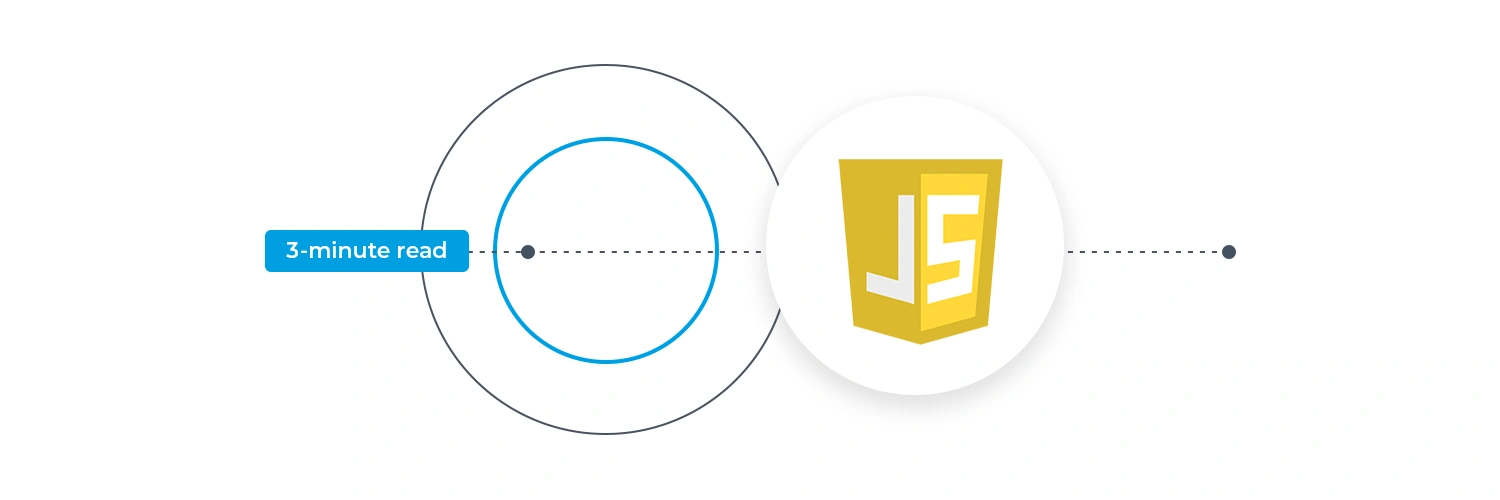# JavaScript Operators

### Author: Daragh Ó Tuama

JavaScript OperatorsJavaScript operators are unique symbols used to carry out actions on operands (values and variables). For instance, 2 + 4; // 6, Here, the operator + performs addition, and the operands are 2 and 4.

This post discusses relational operators and boolean operators in JavaScript.

## Relational Operators in JavaScript

Relational operators are operators that check whether a relationship between two values (like “less-than” or “property-of”) exists and deliver true or false depending on the result.

The following is a list of the JavaScript relational operators.

• greater-than >
• less-than <
• greater-than-or-equal-to >=
• less-than-or-equal-to <=

These comparison operators accept any kind of operand as an argument. Operands that are not integers or strings must be transformed because comparisons can only be made on those types of operands. Following are examples of comparison and conversion:

• They are quantitatively compared if one or both operands are numbers or converted to numbers.
• Both operands are evaluated as strings if they are strings or can be converted to strings.
• The operator tries to convert the string to a number and conduct a numerical comparison if one operand is or transforms to a string and the other operand is or converts to a number. The comparison is false if the string does not represent a number because it converts to NaN in that case.
• JavaScript handles the numerical conversion when an object can be converted into a number or a string. This implies, for instance, that Date objects are numerically compared, and it makes sense to compare two dates to determine which is earlier.
• The comparison operators always yield false if neither of their operands can be effectively transformed to a number or a string.
• The comparison operator always returns false if one or both operands are NaN or converted to NaN.

Remember that string comparison strictly compares each character using its numerical value as determined by the Unicode encoding. The JavaScript comparison operators do not recognise these encoding variations. Instead, they presume that all strings are written in normalised form, even though the Unicode standard permits comparable strings to be encoded in some situations using various character sequences.

Keep in mind that string comparisons are case-sensitive and that all capital letters are “less than” all lowercase letters in the Unicode encoding (at least for the ASCII subset). If you don’t expect it, this rule can have unclear outcomes. For instance, the string “Zoo” is less than the string “aardvark,” according to the operator.

## Boolean Operators in JavaScript

When talking about boolean operators, “and”, “or”, and “not” are the three available operators. They are particularly helpful to developers when creating parts of a complex logic or flow and may be utilised in either a database or coding.

Let’s get a better understanding of how each of these is implemented in JavaScript. We look at:

1. JavasScript OR Operator
2. JavaScript AND Operator
3. JavaScript NOT Operator

### JavaScript OR Operator

In JavaScript, the OR operator is symbolically represented by two vertical lines. Let’s take the following illustration as an example:

Syntax:

``result = a || b; // same as a OR b``

The Boolean operator is only used to assess several Boolean variables in a typical computer language. The expression evaluates and returns true if any of the supplied variables are true; otherwise, it would produce a false value.

### JavaScript AND Operator

The two ampersands && serve as the symbolic representation of the AND operator in JavaScript.

Syntax:

``var result = a && b; // equivalent to a AND b``

The Boolean AND operator is used to test various Boolean operands, just like the OR operator. The expression tests and returns false if any of the supplied variables are false; otherwise, it would return a true value.

Basically, using the AND operator, the following four logical Boolean value combinations are listed:

• False || True i.e False“OR” True // the output will be False
• True || True i.e. True “OR” True // the output will be True
• False || False i.e False“OR” False // the output will be False
• True || False i.e True “OR” False // the output will be False

### JavaScript NOT Operator

The exclamation mark is used as the symbolic representation of the NOT operator in JavaScript.

Syntax:

``var result = ! y;``

The Boolean AND operator, like the OR and AND operators, is exclusively used to test various Boolean operands. Since it returns the inverse of the operand that was supplied to it, it is used to invert the operand’s value.

### 5 Day Coding Challenge – learn JavaScript basics

Code Institute’s free 5 Day Coding Challenge can offer you some insights into HTML, CSS & JS. The best thing about the challenge, other than learning the basics, is that it’ll let you know if you have an aptitude for software development. Register for this weekly challenge through the form below. Alternatively, if you want to learn more about our Full Stack Software Development programme, follow this link